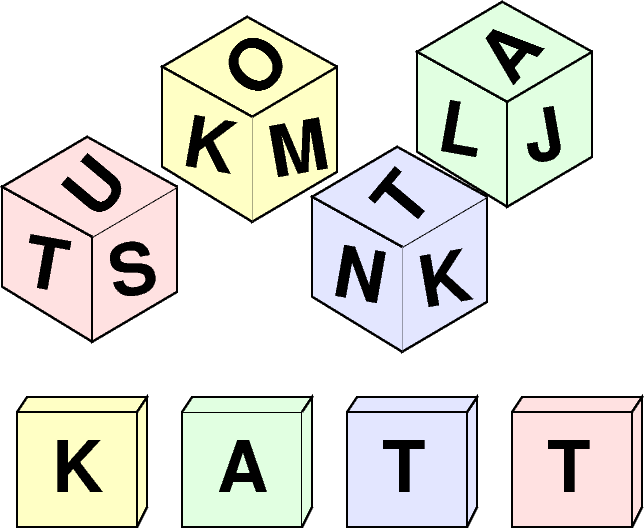시간 제한 메모리 제한 제출 정답 맞은 사람 정답 비율
1 초 1024 MB 2 0 0 0.000%

## 문제

Klara has $N$ dices with letters written on them. Each die has a letter on each of its $K$ sides. By throwing the dice and rearranging them in some arbitrary order, you can make construct a word with $N$ letters.

Write a program to count the number of valid words that can be constructed using Klara's dice. You will get a wordlist, that contains all the $M$ valid $N$-letter words.Figure 1: An illustration of the first example. Since $K = 3$, each die has three sides. You can also write STOL and MASK, but not NATT or KOST.

## 입력

The first line of input contains three space-separated integers $N$, $K$ and $M$.

The next $N$ lines each describe a die. Line $i$ will contain $K$ letters, the letters on the sides of the $i$:th die.

Finally, there will be $M$ lines, the valid words. Each line will contain an $N$-letter word.

All words will only capital letters A-Z.

No letter will appear on more than one side of a die.

## 출력

Your program should print a single integer: the number of valid words that can be written.

## 서브태스크

번호 배점 제한
1 9

$K = 2, N \le 4, M \le 100$

2 9

$K \le 6, N \le 5, M \le 100$

3 12

$K \le 20, N \le 6, M \le 1000$

4 14

$K \le 15, N \le 6, M \le 10\,000$

5 21

$K \le 20, N \le 6, M \le 100\,000$

6 35

$K \le 10, N \le 13, M \le 500$

## 예제 입력 1

4 3 5
TSU
NKT
KMO
LJA
KATT
NATT
STOL
MASK
KOST


## 예제 출력 1

3


## 출처• 문제를 만든 사람: Pär Söderhjelm

## 채점 및 기타 정보

• 예제는 채점하지 않는다.